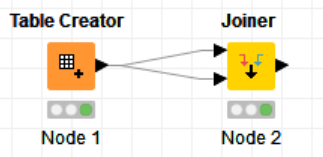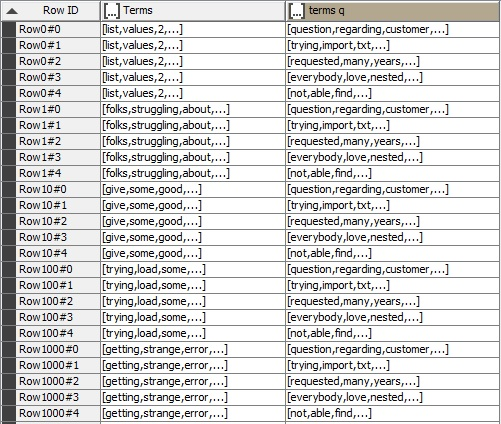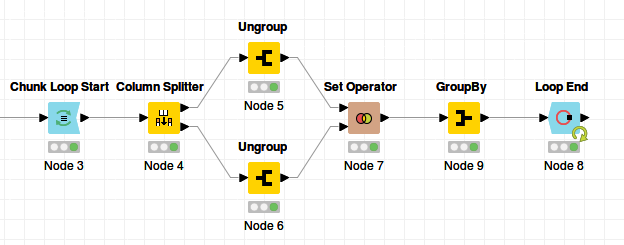# How to find the intersection set of 2 sets

I have 2 collection columns both containing a list of words.
I want to find a list of words which exist in both of these 2 lists.
Example1:
column A: [good, bad, very, apple]
column B: [banana, ball, bad, chair, apple]
The column I want: [bad, apple]

Example2:
column A: [good, bad, very, apple]
column B: [banana, ball, chair]
The column I want: [ ] or missing value (?)

Best,
Armin

Hi Armin,

You just need two nodes:Node 1 is your input data-set with the two columns, Node 2 is a simple join where you select Column A and Column B as the respective joining columns.

For Example1: this will list the value that exist in both data-sets in the first column of the Joiner output.
For Example2: this will create an empty table since there are no matching values.

Cheers,
Medzi

Sorry Medzi, I think I have expressed my question weakly. Let me try again:
I have a table including 2 columns that their type is collection.
That means in each row of the table I have two lists of words and I want to find the intersection of these two lists in each row.
I have tried using Set Operator and Subset Matcher nodes but I couldn’t get my desired result.

Here is an image of the table:PS: I also tried to ungroup and match the terms one by one. But unfortunately the size of the table exceeds my system resources when the Ungroup node is at 4% of the progress.Are you ungrouping the whole table at once? If yes, you can try to ungroup row by row:If that doesn’t work, I think your only option is a java snippet:

``````String[] col1 = \$Terms\$;
String[] col2 = \$terms q\$;

int n1 = col1.length;
int n2 = col2.length;
int n0 = (n1 < n2 ? n1 : n2);

String[] col0 = new String[n0];

int t0 = 0;
for(int t1=0; t1<n1; t1++) {
for(int t2=0; t2<n2; t2++) {
if(col1[t1].equals(col2[t2])) {
col0[t0] = col1[t1];
t0++;
}
}
}

String[] colf = new String[t0];
for(int t1=0; t1<t0; t1++) {
colf[t1] = col0[t1];
}

return colf;
``````

This code can probably be written in a smarter way than above, but it seems to work as long as the terms in the lists are unique.

2 Likes

Ungrouping both columns at once doesn’t result in what I wanted and using 2 Ungroups one after another uses too much resources.

Thank you so much for the code. It seems it’s working.

Cheers,
Armin

@Aswin would you please explain to me what exactly happens if the lists have redundant terms?

Thanks again,
Armin

If “folks” appears 2 times per row in column “Terms” and 3 times per row in “terms q”, your finals list will contain 2x3=6 times “folks”.

If this is not what you want, you can replace the last line in the java code with

``````Set<String> foo = new HashSet<String>(Arrays.asList(colf));
return foo.toArray(new String[foo.size()]);
``````

This will make all terms in the result unique.

1 Like

Thank you so much for the explanation.This topic was automatically closed 7 days after the last reply. New replies are no longer allowed.